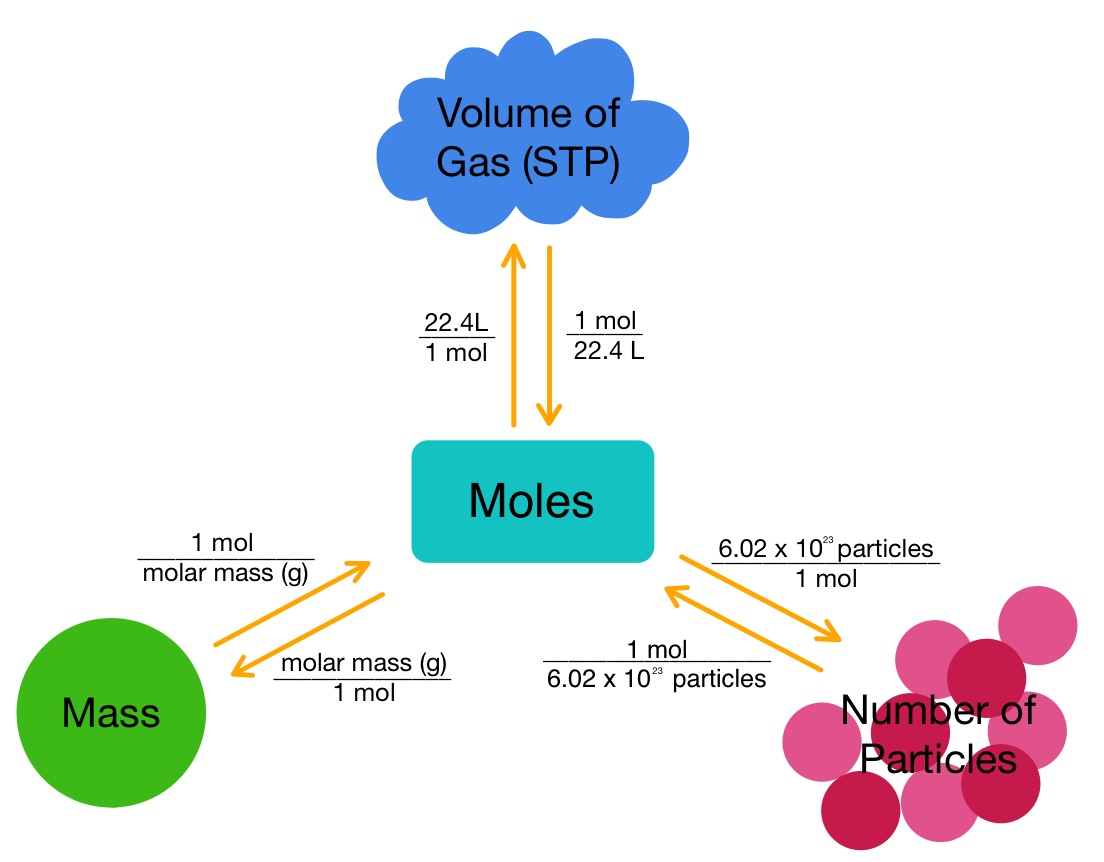# Mole Road Map Study Guide

INTRODUCTION

It is an obvious fact that Frodo Baggins could have never reached Mount Doom without the directions from Gollum or without a perfect map in The Lord of the Rings. Similarly, a map or online instructions are imperial to reach a destination. Mole road maps in chemistry follow a similar pattern. What is the best way to handle a specific sort of calculation? There is a procedure and a set of instructions to assist you.

### MOLE FRACTION

Any measurement has two parts: the quantitative magnitude and the units in which the quantitative magnitude is represented. The magnitude is ‘8’, and the unit is ‘kilogram’ when the mass of a kid is measured to be 8 kilograms. Even one gram of a pure element contains a large number of atoms when interacting with particles at the atomic (or molecular) level. The mole notion is commonly employed in this context. It is largely concerned with the mole unit.

One mole of pure carbon-12 (12C) has a mass of exactly 12 grams and contains 6.02214076*1023 (NA) number of 12C atoms, for instance. The following formula can be used to calculate the number of moles in a pure sample:

``                             n = N/NA``

Here, NA is the Avogadro’s constant, and n is the number of moles of the substance (or elementary entity). N is the total number of elementary entities in the sample.

Let us recall three conversions from previous classes. Remember that n is the number of moles.

• Moles to mass: n × (molar mass )= X grams
• Moles to particles: n × 6.022×1023= X particles
• moles of gas to volume: n (gas) ×22.4 = X liters

At STP (Standard Temperature and Pressure), a simple visual aid to remembering how to convert between moles and volume, moles and mass, and moles and particle count.Source

⇒ At STP, a specific process yields 86.5L of hydrogen gas. What was the total number of moles of hydrogen produced?

Step 1 is to make a list of the known quantities and come up with a plan for solving the problem. Here we know that we have 86.5 L of hydrogen and 1 mol = 22.4 l, and the volume is unknown

Step 2 is to calculate using the conversion factors

86.5 × 1 mol H₂ / 22.4 l H₂ = 2.86 mol of hydrogen.

## CONCLUSION:

1. A mole is the quantity of a substance that includes precisely 6.02214076 * 1023 ‘elementary entities’ of the given substance.
2. The number of moles in a pure sample can be calculated as n = N/NA.
3. Where NA is the Avogadro’s constant and n is the number of moles of the substance.

### FAQs:

1. How do you use the mole map?

A. A mole map can be used by first noting the unknown and known quantities and later using conversion factors to calculate the solution.

2. How do you find the percent composition of a mole?

A.The molecular formula is used to compute the percent composition of a compound: divide the weight of each element found in one mole of the compound by the total molar mass.

We hope you enjoyed studying this lesson and learned something interesting about Mole Town Chemistry! Join our Discord community to get any questions you may have answered and to engage with other students just like you! Don’t forget to download our app to experience our fun VR classrooms – we promise it makes studying much more fun! 😎

## SOURCES:

1. Mole basic concept. https://byjus.com/chemistry/mole-concept-basics/. Accessed 21 Feb 2022